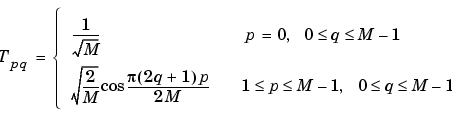Image Processing Toolbox User's GuideThe DCT Transform Matrix

The Image Processing Toolbox offers two different ways to compute the DCT. The first method is to use the function `dct2`. `dct2` uses an FFT-based algorithm for speedy computation with large inputs. The second method is to use the DCT transform matrix, which is returned by the function `dctmtx` and might be more efficient for small square inputs, such as 8-by-8 or 16-by-16. The M-by-M transform matrix `T` is given byFor an M-by-M matrix `A`, `T*A` is an M-by-M matrix whose columns contain the one-dimensional DCT of the columns of `A`. The two-dimensional DCT of `A` can be computed as `B=T*A*T'`. Since `T` is a real orthonormal matrix, its inverse is the same as its transpose. Therefore, the inverse two-dimensional DCT of `B` is given by `T'*B*T`.Discrete Cosine Transform DCT and Image Compression